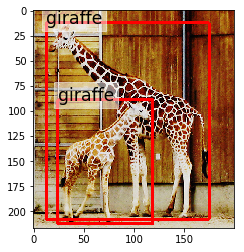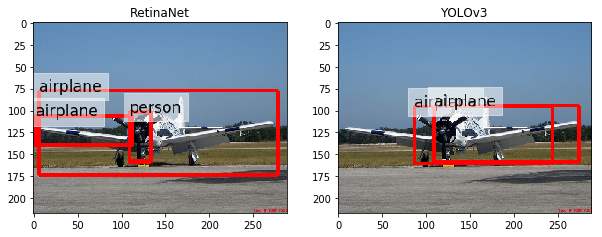# Tutorials¶

## Logging¶

To see logs, use the `logging` API. The logs can be useful for keeping track of what is happening behind the scenes.

```import logging
logging.basicConfig()
logging.getLogger().setLevel('INFO')
```

## Browsing image data¶

```from mira import datasets
coco.show()
```## Augmentation¶

Augmentation can be kind of a pain for object detection sometimes. But `albumentations` makes it pretty easy to build augmentation pipelines and mira uses a protocol to specify how the augmentation should work. Note that, if you use `albumentations`, you must use the ‘pascal_voc’ format. Alternatively, you can use an arbitrary function that adheres to the `mira.core.utils.AugmenterProtocol` protocol.

```from mira import datasets
from mira import core
import albumentations as A

scene = dataset

augmenter = core.augmentations.compose([A.HorizontalFlip(p=1), A.GaussianBlur()])

fig, (ax_original, ax_augmenter) = plt.subplots(ncols=2, figsize=(10, 5))
ax_original.set_title('Original')
ax_augmenter.set_title('Augmented')

scene.show(ax=ax_original)
scene.augment(augmenter).show(ax=ax_augmenter)
```## Basic object detection¶

The example below shows how easy it is to do object detection using the common API for detectors.

```from mira import datasets, detectors
import matplotlib.pyplot as plt

# Load the VOC dataset (use the validation
# split to save time)

# Load FasterRCNN with pretrained layers. It is
# set up to use COCO labels.
detector_faster = detectors.FasterRCNN(pretrained_top=True)

detector_retina = detectors.RetinaNet(pretrained_top=True)

# Pick an example scene
scene = dataset

# Set up side-by-side plots
fig, (ax_retinanet, ax_faster) = plt.subplots(ncols=2, figsize=(10, 5))
ax_retinanet.set_title('EfficientDet')
ax_faster.set_title('FasterRCNN')

# We get predicted scenes from each detector. Detectors return
# lists of annotations for a given image. So we can just replace
# (assign) those new annotations to the scene to get a new scene
# reflecting the detector's prediction.
predicted_retinanet = scene.assign(
annotations=detector_retina.detect(scene.image),
categories=detector_retina.categories
)
predicted_faster = scene.assign(
annotations=detector_faster.detect(scene.image, threshold=0.4),
categories=detector_faster.categories
)

# Plot both predictions. The calls to annotation() get us
# an image with the bounding boxes drawn.
_ = predicted_retinanet.show(ax=ax_retinanet)
_ = predicted_faster.show(ax=ax_faster)
```## Transfer Learning¶

This example inspired by the TensorFlow object detection tutorial.

```import albumentations as A
from mira import datasets, detectors, core

# Load the Oxford pets datasets with a class
# for each breed.

# Load FasterRCNN with pretrained backbone. We'll
# use the annotation configuration for our
detector = detectors.FasterRCNN(
pretrained_top=False,
pretrained_backbone=True,
categories=dataset.categories,
)

# Split our dataset into training, validation,
# and test.
trainval, testing = dataset.train_test_split(
train_size=0.7, test_size=0.3
)
training, validation = trainval.train_test_split(
train_size=0.66, test_size=0.33
)

# Create an augmenter
augmenter = core.augmentations.compose([A.HorizontalFlip(p=1), A.GaussianBlur()])

# Run training job
detector.train(
training=training,
validation=validation,
epochs=1000,
batch_size=8,
augmenter=augmenter,
)
```

## Training on COCO 2014¶

Start by downloading all of the COCO images. If you haven’t already, install `gsutil`, which will make downloading the images a snap.

```curl https://sdk.cloud.google.com | bash
mkdir coco
cd coco
mkdir images
gsutil -m rsync gs://images.cocodataset.org/train2014 images
gsutil -m rsync gs://images.cocodataset.org/val2014 images
gsutil -m rsync gs://images.cocodataset.org/test2014 images
```

Now download the annotations. The following will create an `annotations` folder inside of your `coco` folder.

```curl -LO  http://images.cocodataset.org/annotations/annotations_trainval2014.zip -o annotations_trainval2014.zip
unzip annotations_trainval2014.zip
```

```from mira import datasets

annotations_file='coco/annotations/instances_train2014.json',
image_dir='coco/images'
)
annotations_file='coco/annotations/instances_val2014.json',
image_dir='coco/images'
)
```

And now we can train a detector.

```from mira import detectors

detector = detectors.FasterRCNN(
categories=training.categories
)
detector.train(
training=training,
validation=validation,
epochs=1000
)
```

## Deployment¶

You can deploy your models using TorchServe. The example below exports pretrained models to the MAR format.

```import mira.detectors as md

detector1 = md.FasterRCNN(pretrained_top=True, backbone="resnet50")
detector1.to_torchserve("fastrcnn")
detector2 = md.RetinaNet(pretrained_top=True)
detector2.to_torchserve("retinanet")
```

The above will generate `fastrcnn.mar` and `retinanet.amr` in the `model-store` directory. You can then use those models with TorchServe using:

```torchserve --start --model-store model-store --models retinanet=retinanet.mar,fastrcnn=fastrcnn.mar
```

Then you can do inference in TorchServe using something like the following.

```# Get responses from server.
with open("path/to/image.jpg", "rb") as f:
prediction1 = requests.post("http://localhost:8080/predictions/fastrcnn", data=data).json()
prediction2 = requests.post("http://localhost:8080/predictions/retinanet", data=data).json()
categories = mc.Categories(list(set(p["label"] for p in prediction1 + prediction2)))
scene1, scene2 = [
mc.Scene(
image=filename,
categories=categories,
annotations=[
mc.Annotation(
x1=p["x1"],
y1=p["y1"],
x2=p["x2"],
y2=p["y2"],
category=categories[p["label"]],
score=p["score"]
) for p in prediction
]
) for prediction in [prediction1, prediction2]
]
```

You can stop the server using `torchserve --stop`. Note that to restart the server, you should delete the `logs` directory that `torchserve` creates as it stores cached state that you may not want.